##Rectangular Hyperbola

Rectangular hyperbola (x-a)*(y-b) = a*b. The hyperbola is constructed through the tool [Conics\Hyperbola\Rectangular Hyperbola], by setting its center at P(a,b) and a vertex of it at C. C is a point depending on P and is constructed via the [RH-trans], which is a transformation, calculating C for every given P. The script for this transformation is contained in the file [EUC_Scripts\EUC_Transforms\RectHyp.txt]. The figure has a nice interpretation of a geometric relation, explained in the file RectHypeRelation.html .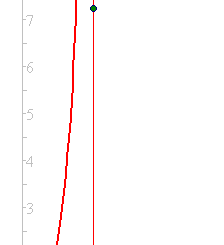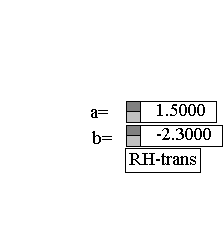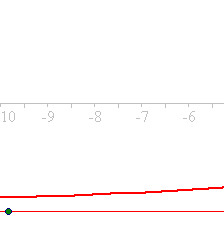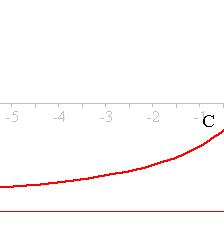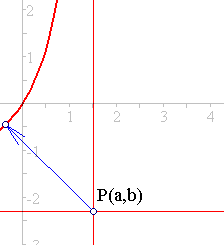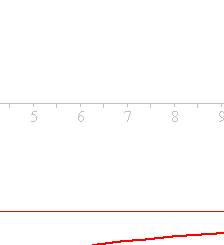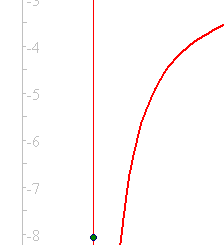AsymptoticTriangle.html
Chasles_Steiner.html
Chasles_Steiner_Envelope.html
HomographicRelation.html
HomographicRelationExample.html
Hyperbola.html
HyperbolaAsymptoticProperty.html
HyperbolaAsymptotics.html
HyperbolaRectangular.html
Line_Homography.html
RectangularAsProp.html
RectHypeRelation.html